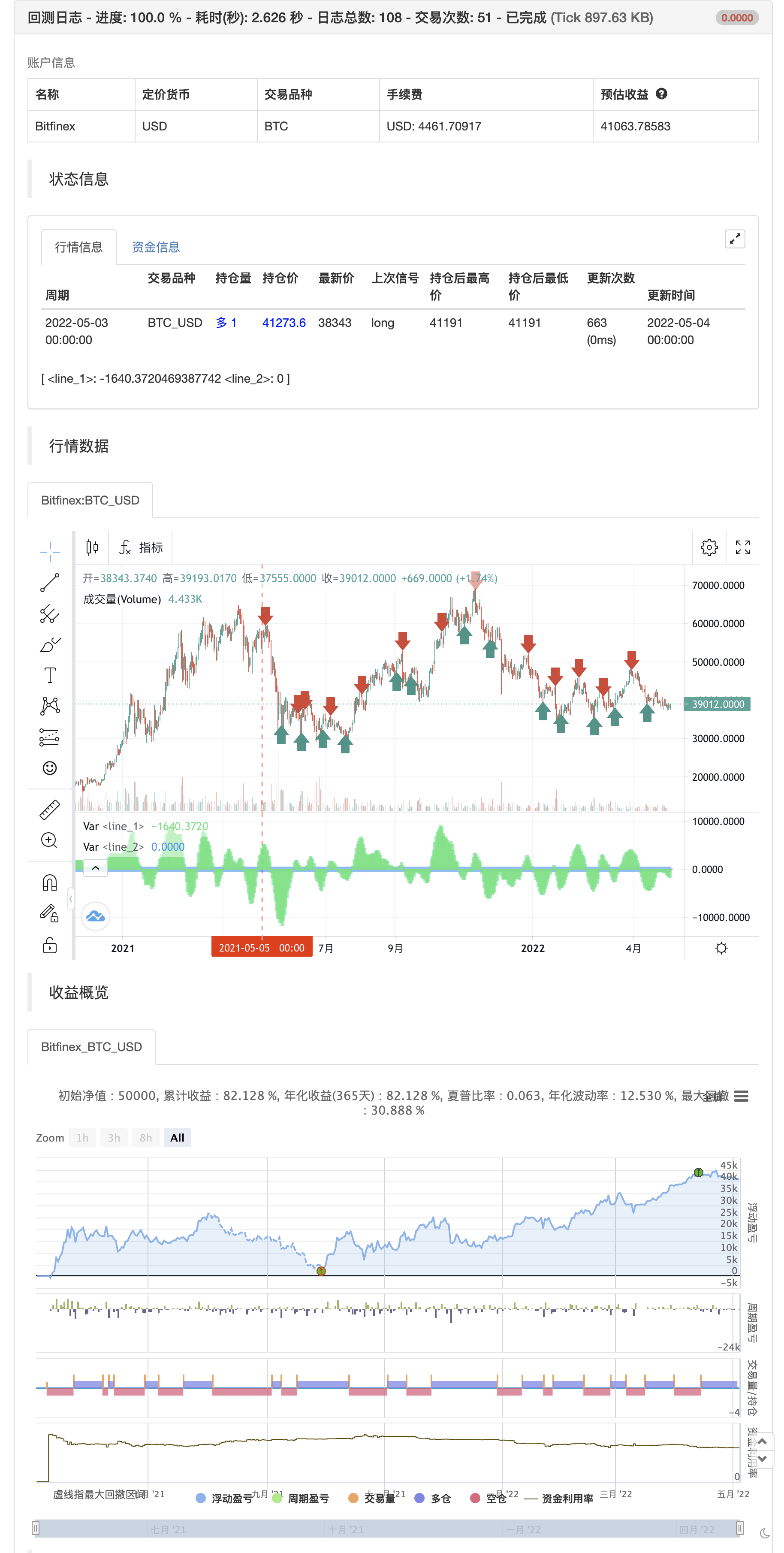# Squeeze Momentum Indicator

Author: 张超, Date: 2022-05-06 17:19:10
Tags: sma

This is a derivative of John Carter’s “TTM Squeeze” volatility indicator, as discussed in his book “Mastering the Trade” (chapter 11).

Black crosses on the midline show that the market just entered a squeeze ( Bollinger Bands are with in Keltner Channel). This signifies low volatility , market preparing itself for an explosive move (up or down). Gray crosses signify “Squeeze release”.

Mr.Carter suggests waiting till the first gray after a black cross, and taking a position in the direction of the momentum (for ex., if momentum value is above zero, go long). Exit the position when the momentum changes (increase or decrease — signified by a color change). My (limited) experience with this shows, an additional indicator like ADX / WaveTrend, is needed to not miss good entry points. Also, Mr.Carter uses simple momentum indicator , while I have used a different method (linreg based) to plot the histogram.```/*backtest
start: 2021-05-05 00:00:00
end: 2022-05-04 23:59:00
period: 1d
basePeriod: 1h
exchanges: [{"eid":"Bitfinex","currency":"BTC_USD"}]
*/

//
// @author LazyBear
// List of all my indicators: https://www.tradingview.com/v/4IneGo8h/
//
study(shorttitle = "SQZMOM_LB", title="Squeeze Momentum Indicator [LazyBear]", overlay=false)

length = input(20, title="BB Length")
mult = input(2.0,title="BB MultFactor")
lengthKC=input(14, title="KC Length")
multKC = input(1.5, title="KC MultFactor")

useTrueRange = input(true, title="Use TrueRange (KC)",defval=true)

// Calculate BB
source = close
basis = ta.sma(source, length)
dev = multKC * ta.stdev(source, length)
upperBB = basis + dev
lowerBB = basis - dev

// Calculate KC
ma = ta.sma(source, lengthKC)
range = useTrueRange ? ta.tr : (high - low)
rangema = ta.sma(range, lengthKC)
upperKC = ma + rangema * multKC
lowerKC = ma - rangema * multKC

sqzOn  = (lowerBB > lowerKC) and (upperBB < upperKC)
sqzOff = (lowerBB < lowerKC) and (upperBB > upperKC)
noSqz  = (sqzOn == false) and (sqzOff == false)

val = ta.linreg(source  -  math.avg(math.avg(ta.highest(high, lengthKC), ta.lowest(low, lengthKC)),ta.sma(close,lengthKC)),
lengthKC,0)

bcolor = iff( val > 0,
iff( val > nz(val), color.lime, color.green),
iff( val < nz(val), color.red, color.maroon))

scolor = noSqz ? color.blue : sqzOn ? color.black : color.gray
plot(val, color=bcolor, style=plot.style_histogram, linewidth=4)
plot(0, color=scolor, style=plot.style_cross, linewidth=2)

if val >0 and val < nz(val)
strategy.entry("entry short", strategy.short)
else if val <0 and val > nz(val)
strategy.entry("entry long", strategy.long)

```

Related

More